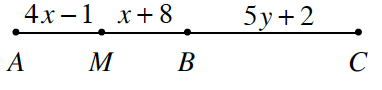Home > CCG > Chapter Ch7 > Lesson 7.2.2 > Problem7-65

7-65.Point $M$ is the midpoint of $\overline{AB}$ and $B$ is the midpoint of $\overline{AC}$. What are the values of $x$ and $y$? Show all work and reasoning.

How do $AM$ and $MB$ relate to each other if $M$ is the midpoint? How can you use this to solve for $x$?

Once you have found $x$, use a similar method to find $y$.